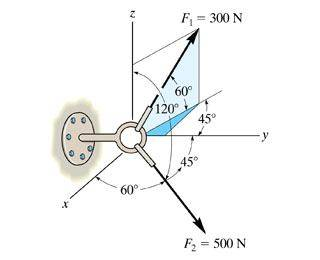# Expressing force in cartesian vector form

## Homework StatementExpress F1 and F2 in cartesian vector form

## The Attempt at a Solution

I feel fairly confident in my work for F2 but F1 not so much. Especially the j component of F1. I assume my F(1x) component runs from the z axis to the tip of F1 and my F(1k) component runs from the tip of F1 to the line below (which forms the light blue triangle). F(1y) runs along the y axis and forms the base of the dark blue triangle (I think). Any help would be much appreciated!

F(1x)= -300cos60 = -150lb
F(1y) = 300cos60sin45 = 106lb
F(1z) = 300sin60 = 260lb
F1 = -150i + 106J + 260k

F2 = 50cos60i + 500cos45j + 500cos120 = 250i + 354j - 250k

TSny
Homework Helper
Gold MemberI assume my F(1x) component runs from the z axis to the tip of F1
I don't think this is correct.

All of your other components for F1 and all of your components for F2 look correct to me.

Are you saying my F(1x) does not look correct?

SammyS
Staff Emeritus
Homework Helper
Gold Member

## Homework StatementExpress F1 and F2 in cartesian vector form

## The Attempt at a Solution

I feel fairly confident in my work for F2 but F1 not so much. Especially the j component of F1. I assume my F(1x) component runs from the z axis to the tip of F1 and my F(1k) component runs from the tip of F1 to the line below (which forms the light blue triangle). F(1y) runs along the y axis and forms the base of the dark blue triangle (I think). Any help would be much appreciated!

F(1x)= -300cos60 = -150lb
F(1y) = 300cos60sin45 = 106lb
F(1z) = 300sin60 = 260lb
F1 = -150i + 106J + 260k

F2 = 50cos60i + 500cos45j + 500cos120 = 250i + 354j - 250k
It looks to me that the base of the F1 parallelogram (rectangle) in the figure lies in the x-y plane and makes an angle of 45° with the y-axis.

The F1 makes an angle of 60° with the base of the parallelogram (rectangle).

I agree. What part of my work do you not think is correct?

SammyS
Staff Emeritus
Homework Helper
Gold Member
I agree. What part of my work do you not think is correct?
The magnitude of your vector is not 300 N.

TSny
Homework Helper
Gold Member
Are you saying my F(1x) does not look correct?
F(1x)= -300cos60 = -150lb
Yes, I don't agree with your result for F1x. I think it should be similar to your calculation for F1y.
F(1y) = 300cos60sin45 = 106lb
For your calculation of F1y, why did you use the sine of 45 rather than the cosine of 45?

I figured the cosine would give the base of the parallelogram. I thought I needed the base of the dark blue triangle along the y axis to give the y component. I though 300 cos30 was the hypotenuse of the dark blue triangle.

TSny
Homework Helper
Gold Member
I thought I needed the base of the dark blue triangle along the y axis to give the y component.
Yes. But isn't the base of the dark blue triangle (along the y axis) the "side adjacent" to the angle marked 45 degrees in the triangle? So, I would think you would use the cosine rather than the sine of 45 degrees. (Of course they happen to be equal for 45 degrees, but if it had been 40 degrees you would need to choose the correct trig function.)
I though 300 cos30 was the hypotenuse of the dark blue triangle.
Yes, that's right. Isn't the x component of F1 equal to the other leg of the dark blue triangle?

Maybe my old eyes aren't seeing the diagram correctly.

SammyS
Staff Emeritus
Homework Helper
Gold Member
Yes, that's right. Isn't the x component of F1 equal to the other leg of the dark blue triangle?
I agree, (except for the sign).

•TSny
TSny
Homework Helper
Gold Member
I agree, (except for the sign).
Yes. The sign needs to be considered!

So, F(1x) = -300cos60sin45
F(1y) = 300cos60cos45
F(1z) = 300sin60